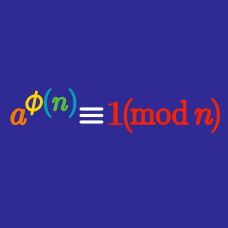Number Theory

# Euler's Theorem: Level 3 Challenges

How many positive integers $$n>1$$ evenly divide $$a^{13}-a$$ for all positive integers $$a?$$

Find the sum of all prime numbers $$p$$ such that $$p|\underset { p }{ \underbrace { 111\dots 1 } }$$.

$\Huge {\color{blue}9}^{{\color{green}8}^{{\color{red}7}^{{\color{brown}6} ^{\color{magenta}5}}}}$

What are the last two digits when this integer fully expanded out?

Let $$P$$ be product of all positive integers less than 720 which are relatively prime to 720. What is the remainder when $$P^2$$ is divided by 720?

Find the tens digit of $\Large 2014^{2014^{2014}}.$

×

Problem Loading...

Note Loading...

Set Loading...